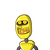# In Fig. AB= AC and AD is the bisector ofZBAC.State three pairs of equal partsin triangles ADB and ADC.(ii)

In Fig. AB= AC and AD is the bisector of
ZBAC.
State three pairs of equal parts
reasons
Is B= 2C? Give reasons.​

### 1 thought on “In Fig. AB= AC and AD is the bisector of<br />ZBAC.<br />State three pairs of equal parts<br />in triangles ADB and ADC.<br />(ii)”

1.i) Three pair of equal parts are:

BD = CD ( as d is the mid point of BC)

AB = AC (given in the question)

ii) Now,

by SSS Congruency rule,